Question

# The hypothesis test for the proportions is calculated using a z test statistic since proportions are...

The hypothesis test for the proportions is calculated using a z test statistic since proportions are based on a binomial distribution. True or False

Given statement is True

Because in case of proportion test we consider number of success (x) out of (n) trials and p is ratio of this two ,so in this case we consider binomial distribution threfore the hypothesis test for proportion is calculated using a z test statistic since proportion are based on a binomial distribution.

,

Dear student,
I am waiting for your feedback. I have given my 100% to solve your queries. If you are satisfied by my given answer. Can you please like it☺

Thank You!!!

#### Earn Coins

Coins can be redeemed for fabulous gifts.

Similar Homework Help Questions
• ### True or False The model used for hypothesis testing of proportions is called a two-proportion z-test....

True or False The model used for hypothesis testing of proportions is called a two-proportion z-test. True or False Beta (β) is the significance level which determines if the null hypothesis is rejected. True or False In business, the p-value is one of several factors in determining whether or not to reject a null hypothesis. True or False We could make a decision to reject a null hypothesis on a very low p-value or a very high z score.

• ### Question 8 2 pts When calculating a t-test statistic in a hypothesis test, it is based...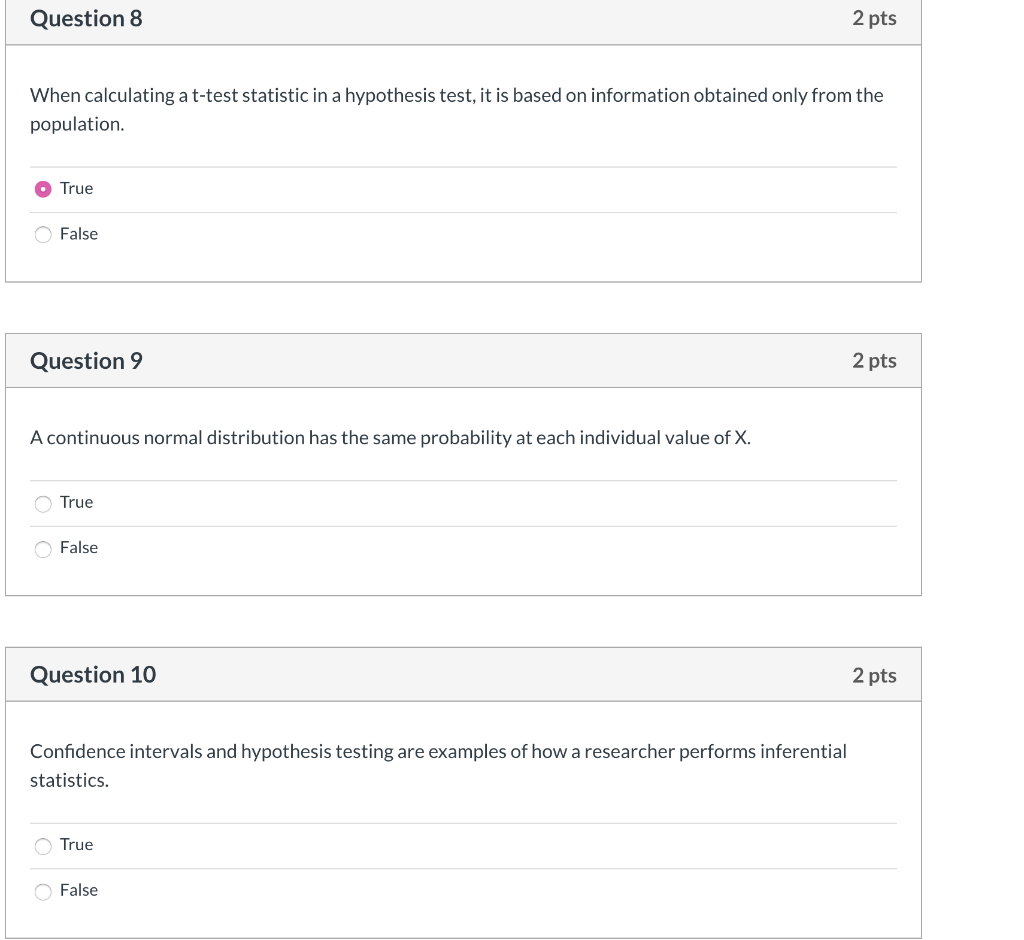Question 8 2 pts When calculating a t-test statistic in a hypothesis test, it is based on information obtained only from the population. True False Question 9 2 pts A continuous normal distribution has the same probability at each individual value of X. True False Question 10 2 pts Confidence intervals and hypothesis testing are examples of how a researcher performs inferential statistics. True False

• ### When conducting a hypothesis test concerning the population proportion, the value of the test statistic is calculated as ____________.

When conducting a hypothesis test concerning the population proportion, the value of the test statistic is calculated as ____________.rev: 07_06_2018_QC_CS-130873Multiple Choicez=x−−μoσ/n√tdf=x−−μos/n√z=p−−popo(1−po)n√tdf=p−−popo(1−po)n√

• ### A P-value of 0.12 is calculated on a hypothesis test with a significance level set at...

A P-value of 0.12 is calculated on a hypothesis test with a significance level set at 0.01. Which of the following is the correct conclusion for the test? a. Claim the null hypothesis is true b. Fail to reject the null hypothesis c. Reject the null hypothesis d. Claim the alternative hypothesis is true Which of the following are not requirements for using the t-distribution for a hypothesis test concerning μ? (More than one answer may be correct.) a. Sample...

• ### When the necessary conditions are met, a two-tailed test is being conducted to test the difference...

When the necessary conditions are met, a two-tailed test is being conducted to test the difference between two population proportions. The two sample proportions are p1hat=0.052 and p2hat=0.023 and the standard error of the sampling distribution of p1-p2 is 0.0085. The calculated value of the test statistic will be z = 3.41. A. True B. False

• ### Identify the null​ hypothesis, alternative​ hypothesis, test​ statistic, P-value, conclusion about the null​ hypothesis, and final...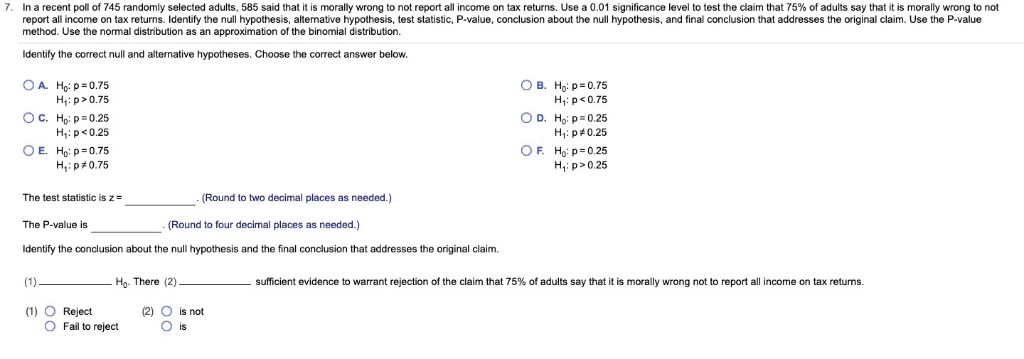Identify the null​ hypothesis, alternative​ hypothesis, test​ statistic, P-value, conclusion about the null​ hypothesis, and final conclusion that addresses the original claim. Use the​ P-value method. Use the normal distribution as an approximation of the binomial distribution. 7. In a recent poll of 745 randomly selected adults, 585 said that it is morally wrong to not report all income on tax returns. Use a 0.01 significance level report all income on tax returns. Identify the null hypothesis, altemative hypothesis, test...

• ### 3. Testing a population mean The test statistic (Chapter 11) Aa Aa You conduct a hypothesis...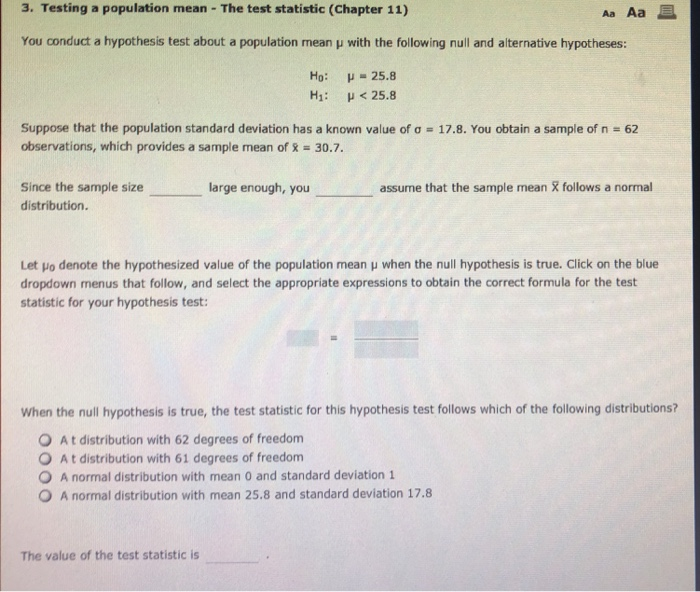3. Testing a population mean The test statistic (Chapter 11) Aa Aa You conduct a hypothesis test about a population mean u with the following null and alternative hypotheses: Ho: u-25.8 H1: <25.8 Suppose that the population standard deviation has a known value of a observations, which provides a sample mean of % 30.7. 17.8. You obtain a sample of n =62 Since the sample size large enough, you assume that the sample mean X follows a normal distribution. Let...

• ### When performing a hypothesis test on the difference between 2 population proportions, the 2-test statistic equation...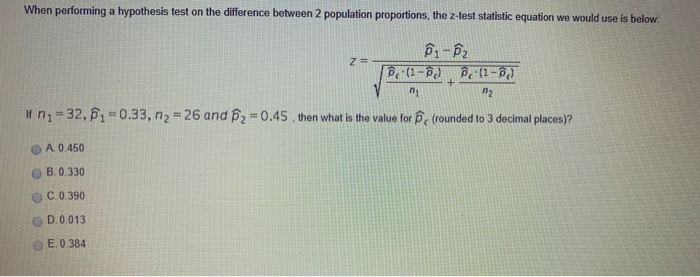When performing a hypothesis test on the difference between 2 population proportions, the 2-test statistic equation we would use is below. 51-52 Poe (1-ộc) , (1-6] If n = 32, 0.33, n2 = 26 and 2=0.45, then what is the value for pc (rounded to 3 decimal places)? A. 0.450 B.0.330 C.0 390 D.0.013 E. 0.384

• ### How do you know if a hypothesis test is testing the claim between 2 population proportions or 2 means? How is the test...

How do you know if a hypothesis test is testing the claim between 2 population proportions or 2 means? How is the test statistic for a claim about 2 population means, independent samples, standard deviations unknown, similar to the test statistic for 1 population mean, standard deviation unknown? If the difference between the 2 population means is not significant, which means the test statistic falls within the "usual" area of the distribution, what is the decision about the null hypothesis?...

• ### I spefically need to see how the test statistic and critical value is calculated. Test the...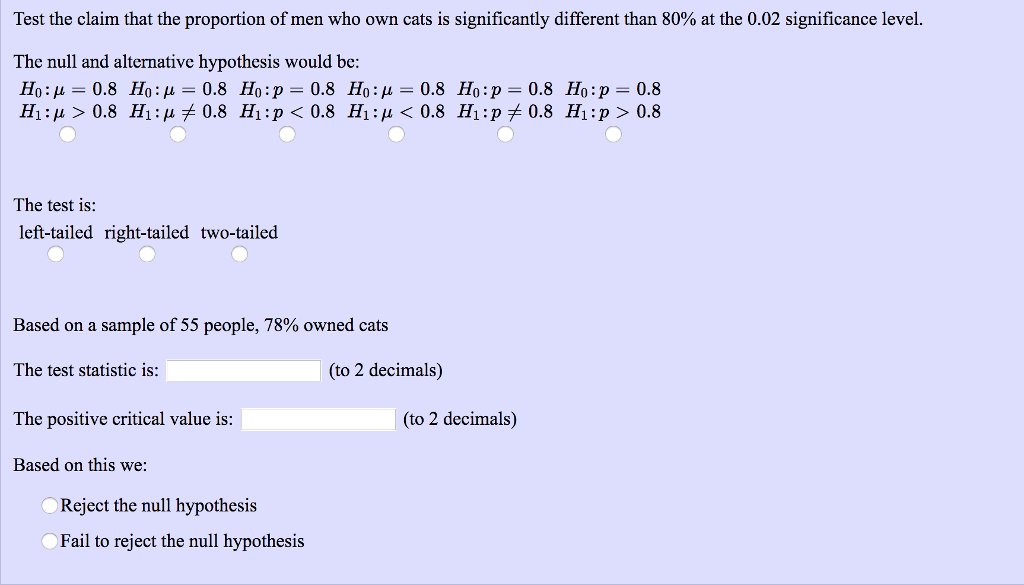I spefically need to see how the test statistic and critical value is calculated. Test the claim that the proportion of men who own cats is significantly different than 80% at the 0.02 significance level. The null and alternative hypothesis would be: The test is: left-tailed right-tailed two-tailed Based on a sample of 55 people, 78% owned cats The test statistic is: (to 2 decimals) The positive critical value is: (to 2 decimals) Based on this we: Reject the null...